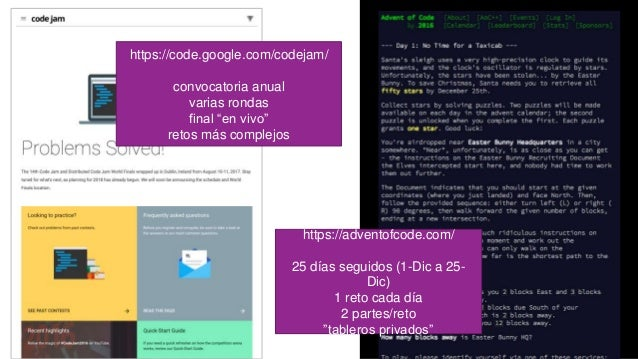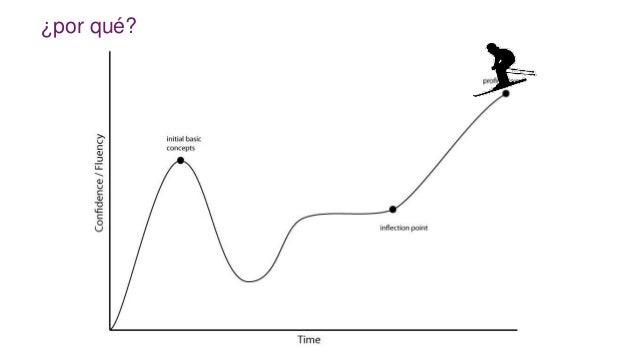Successfully reported this slideshow.You’ve finished this document.
Upcoming SlideShare
Next

ofUpcoming SlideShare
Next

Share

# Retos de Programación en Python

Solución a 4 retos de programación de Advent of Code y Google Code Jam para ilustrar construcciones pythónicas de código y algunas utilidades poco conocidas del lenguaje.

See all

See all

### Retos de Programación en Python

1. 1. Retos de Programación y Estructuras de datos de Python Alicia Pérez Javier Abadía
2. 2. https://code.google.com/codejam/ convocatoria anual varias rondas final “en vivo” retos más complejos https://adventofcode.com/ 25 días seguidos (1-Dic a 25- Dic) 1 reto cada día 2 partes/reto ”tableros privados”
3. 3. ¿por qué?
4. 4. AoC - Día 6 https://adventofcode.com/2016/day/6
5. 5. AoC - Día 6 messages = """ eedadn drvtee eandsr raavrd atevrs tsrnev sdttsa rasrtv nssdts ntnada … """  https://adventofcode.com/2016/day/6  convertir las columnas en listas → transponer  contar la frecuencia de las letras  quedarse con el máximo de cada columna
6. 6. transponer filas a columnas messages = """ eedadn drvtee eandsr … """ messages = messages.strip().split('n'); ncolumns = len(messages) assert all(len(message) == ncolumns for message in messages) # columns = [[]] * ncolumns # bad!! columns = [[] for i in range(ncolumns)] for i in range(ncolumns): for message in messages: columns[i].append(message[i])
7. 7. zip(), iteración en paralelo messages = """ eedadn drvtee eandsr … """ messages = messages.strip().split('n'); ncolumns = len(messages) columns = [[] for i in range(ncolumns)] for message in messages: for char, column in zip(message, columns): column.append(char)
8. 8. comprensiones de listas messages = """ eedadn drvtee eandsr … """ messages = messages.strip().split('n'); ncolumns = len(messages) columns = [[] for i in range(ncolumns)] for i, column in enumerate(columns): column = [message[i] for message in messages] # o también columns = [[message[i] for message in messages] for i in range(ncolumns)]
9. 9. bum! messages = """ eedadn drvtee eandsr … """ messages = messages.strip().split('n'); columns = zip(*messages)
10. 10. contar # bad counting example counts = {} for char in column: if not char in counts: counts[char] = 1 else: counts[char] += 1 sorted_counts = sorted( counts.items(), key=itemgetter(1) ) most_common = sorted_counts[-1] # slightly better counting example counts = defaultdict(int) for char in column: counts[char] += 1 sorted_counts = sorted( counts.items(), key=itemgetter(1) ) most_common = sorted_counts[-1]
11. 11. ‘most common’ → collections.Counter >>> from collections import Counter >>> Counter('abracadabra') Counter({'a': 5, 'r': 2, 'b': 2, 'c': 1, 'd': 1}) >>> Counter('abracadabra').most_common() [('a', 5), ('r', 2), ('b', 2), ('c', 1), ('d', 1)]
12. 12. combinar los más comunes solution = "" for column in columns: solution += Counter(column).most_common() solution = ''.join(Counter(c).most_common() for c in columns)
13. 13. ¿qué hemos aprendido?  enumerate(), zip(), defaultdict()  split(), join()  comprensiones  collections.Counter() messages = messages.strip().split('n'); columns = zip(*messages) solution = ''.join(Counter(c).most_common() for c in columns) print solution
14. 14. AoC - Día 1 https://adventofcode.com/2016/day/1
15. 15. AoC - Día 1  https://adventofcode.com/2 016/day/1  parsear las instrucciones  representar las posiciones y direcciones  aplicar las instrucciones  calcular la distancia Manhattan input = """ L2, L5, L5, R5, L2, L4, R1, R1, L4, R2, R1, … """ (0, 0) hacia el Norte N S W E 2 5 5 (3, -5) → dist=8
16. 16. parsear la entrada input = """ L2, L5, L5, R5, L2, L4, R1, R1, L4, R2, R1, … """ def solve(input): input = [s.strip() for s in input.split(',')] direction = N pos = Vector(0, 0) for step in input: instruction = Instruction(LEFT if step == 'L' else RIGHT, int(step[1:])) direction = turn(direction, instruction.side) for _ in range(0, instruction.advance): pos = advance(pos, directions[direction]) return pos ['L2', 'L5', 'L5', 'R5’, …] from collections import namedtuple Instruction = namedtuple('Instruction', "side advance") t = Instruction(-1, 10) t == -1 t.side == -1 t == 10 t.advance == 10
17. 17. Vector = namedtuple('Vector', "x y") directions = { N: Vector(0, 1), # equiv. Vector(x=0, y=1) E: Vector(1, 0), S: Vector(0, -1), W: Vector(-1, 0), } def advance(pos, direction): delta = directions[direction] return Vector(pos.x + delta.x, pos.y + delta.y) # return Vector(*map(sum, zip(pos, delta))) representar posiciones y direcciones # directions N = 0 # clockwise E = 1 S = 2 W = 3 # sides RIGHT = +1 LEFT = -1 def turn(direction, side): return (direction + side) % 4 assert turn(N, RIGHT) == E assert turn(N, LEFT) == W assert turn(S, RIGHT) == W assert turn(S, LEFT) == E … # N # | # W --+-- E # | # S
18. 18. aplicar las instrucciones y calcular distancia def solve(input): input = [s.strip() for s in input.split(',')] direction = N pos = Vector(0, 0) for step in input: instruction = Instruction(LEFT if step == 'L' else RIGHT, int(step[1:])) direction = turn(direction, instruction.side) for _ in range(0, instruction.advance): pos = advance(pos, direction) return pos if __name__ == '__main__': input = """ … """ pos = solve(input) dist = abs(pos) + abs(pos) print "distance to (0,0) = %d" % (dist,)
19. 19. complicación (2ª parte)  si pasas dos veces por el mismo sitio, terminamos def solve(input): input = [s.strip() for s in input.split(',')] direction = N pos = Vector(0, 0) already_visited = {pos} for step in input: instruction = Instruction(LEFT if step == 'L' else RIGHT, int(step[1:])) direction = turn(direction, instruction.side) for _ in range(0, instruction.advance): pos = advance(pos, direction) if pos in already_visited: return pos already_visited.add(pos) return pos # no se ha repetido ninguna posición
20. 20. hay gente muy PRO def solve(input): input = [s.strip() for s in input.split(',')] direction = 0+1j # (0,1) pos = 0+0j # (0,0) already_visited = {pos} for step in input: side, advance = step, int(step[1:]) direction *= 1j if side == 'L' else -1j for _ in range(advance): pos += direction if pos in already_visited: return pos already_visited.add(pos) return pos if __name__ == '__main__': input = """ … """ pos = solve(input) dist = abs(pos.real) + abs(pos.imag) print "distance to (0,0) = %d" % (dist,) http://mathworld.wolfram.com/Rotation.html N S W E eje real ejeimaginario números complejos operaciones con números complejos • suma: 3+5j + 2-4j = 5+1j • rotación: • 90º izquierda = mult por 0+1j • 90º derecha = mult por 0-1j (2,4) = 2+4j
21. 21. ¿qué hemos aprendido?  strip(), split()  namedtuples()  string slicing  números complejos  assert como test unitarios sencillos  set()
22. 22. Code Jam Qualification Round 2016 Prob B https://code.google.com/codejam/contest/6254486/dashboard#s=p1
23. 23. Code Jam, Qualification Round 2016, Prob B - → + 1 -+ → ++ 1 +- → -- ++ 2 +++ → 0 --+- → +++- ---- ++++ 3
24. 24. def BFS(S): visited, queue = set(), [<initial_state>] while queue: depth, current = queue.pop(0) if is_solution(current): return depth if current not in visited: visited.add(current) <add possible states to queue> return None optimización? BFS
25. 25. def flip(S, i): top_pankakes = ['+' if p == '-' else '-' for p in reversed(S[0:i])] return ''.join(top_pankakes) + S[i:] assert flip('+--+', 1) == '---+' assert flip('+--+', 2) == '-+-+' assert flip('+--+', 3) == '++-+' assert flip('+--+', 4) == '-++-' dando la vuelta a las tortitas
26. 26. def shorten(S): return ''.join(ch for ch, _ in itertools.groupby(S)) def solve(S): visited, queue = set(), [(0, shorten(S))] while queue: depth, current = queue.pop(0) if current.count('+') == len(current): return depth if current not in visited: visited.add(current) for i in range(1, len(current)+1): flipped = shorten(flip(current, i)) if flipped not in visited: queue.append((depth+1, flipped)) return None implementación BFS
27. 27. def minimumFlips(pancakes): groupedHeight = 1 + pancakes.count('-+') + pancakes.count('+-') if pancakes.endswith('-'): return groupedHeight else: return groupedHeight - 1 tiene truco! - → + 1 -+ → ++ 1 +- → -- ++ 2 +++ → 0 --+- → +++- ---- ++++ 3
28. 28. AoC - Día 9 http://adventofcode.com/2016/day/9
29. 29. AoC - Día 9  https://adventofcode.com/2016/day/9 ADVENT ADVENT A(1x5)BC ABBBBBC (3x3)XYZ XYZXYZXYZ (6x1)(1x3)A (1x3)A (6x1)(1x3)A AAA X(8x2)(3x3)ABCY X(3x3)ABC(3x3)ABCY X(8x2)(3x3)ABCY XABCABCABCABCABCABC Y (27x12)(20x12)(13x14)(7x10)(1x12)A longitud 241920 (25x3)(3x3)ABC(2x3)XY(5x2) PQRSTX(18x9)(3x2)TWO(5x7)SEVEN longitud 445
30. 30. unos casos de prueba def do_test(lines): for tc in lines.strip().split('n'): compressed, expected = tc.strip().split(',') uncompressed = uncompress(compressed) assert uncompressed == expected, "bad result %s, expected %s" % (uncompressed, expected) test_cases = """ ADVENT,ADVENT A(1x5)BC,ABBBBBC (3x3)XYZ,XYZXYZXYZ … """
31. 31. A(1x5)BC ADVENT X(8x2)(3x3)ABCY def uncompress(c): m = re.match(r'([^()]*)((d+)x(d+))(.*)', c) if not m: return len(c) pre, length, reps, rest = m.groups() length, reps = int(length), int(reps) return len(pre) + uncompress(rest[:length]) * reps + uncompress(rest[length:]) A(1x5)BC pre, length, reps, rest ([^()]*)((d+)x(d+))(.*) ABBBBBC X(8x2)(3x3)ABCY pre, length, reps, rest ([^()]*)((d+)x(d+))(.*) XABCABCABCABCABCABCY regex
32. 32. regex - verbose r = re.compile(r""" ([^()]*) # pre ((d+)x(d+)) # ( length x reps ) (.*) # rest """, re.VERBOSE)
33. 33.  assert  recursividad  slicing  regex  regex + verbose ¿qué hemos aprendido?
34. 34. resumen  zip, enumerate  tuple, set, dict  collections  namedtuple, Counter, defaultdict  itertools  groupby  product, permutations  slicing [:], join, split  assert  regex python es MUY expresivo tiene su propio “acento” nunca paras de aprender practicar, practicar, practicar
35. 35. trucos  los tipos de problemas se repiten  librería de funciones de uso común  vectores  lectura de la entrada  strip(), split()  pensar/escribir/pintar antes de escribir código  casos de prueba pequeños  assert  fuerza bruta, no suele funcionar  pero da ideas de cómo resolverlo bien
36. 36. Referencias  Cracking the Code Interview, Gayle Laakmann McDowel  Fluent Python, Luciano Ramalho  Python Essential Reference, David M. Beazley  reddit
37. 37. Alicia Pérez Javier Abadía ¡Gracias!
•Aug. 9, 2018

Total views

1,059

On Slideshare

0

From embeds

0

Number of embeds

3

0

Shares

0